# pylops.signalprocessing.Convolve1D#

class pylops.signalprocessing.Convolve1D(dims, h, offset=0, axis=-1, method=None, dtype='float64', name='C')[source]#

1D convolution operator.

Apply one-dimensional convolution with i) a compact filter (shorter than input signal) or ii) an extended filter (larger than input signal) to model (and data) along an axis of a multi-dimensional array.

Parameters
dims

Number of samples for each dimension of the model

hnumpy.ndarray

1d filter to be convolved to input signal

offsetint

Index of the center of the filter

axisint, optional

New in version 2.0.0.

Axis along which convolution is applied

methodstr, optional

Method used to calculate the convolution (direct, fft, or overlapadd). Note that only direct and fft are allowed when dims=None, whilst fft and overlapadd are allowed when dims is provided.

dtypestr, optional

Type of elements in input array.

namestr, optional

New in version 2.0.0.

Name of operator (to be used by pylops.utils.describe.describe)

Raises
ValueError

If offset is bigger than len(h) - 1

NotImplementedError

If method provided is not allowed

Notes

The Convolve1D operator applies convolution between the input signal $$x(t)$$ and a filter kernel $$h(t)$$ in forward model:

$y(t) = \int\limits_{-\infty}^{\infty} h(t-\tau) x(\tau) \,\mathrm{d}\tau$

This operation can be discretized as follows

$y[n] = \sum_{m=-\infty}^{\infty} h[n-m] x[m]$

as well as performed in the frequency domain.

$Y(f) = \mathscr{F} (h(t)) * \mathscr{F} (x(t))$

Convolve1D operator uses scipy.signal.convolve that automatically chooses the best domain for the operation to be carried out for one dimensional inputs. The fft implementation scipy.signal.fftconvolve is however enforced for signals in 2 or more dimensions as this routine efficently operates on multi-dimensional arrays.

As the adjoint of convolution is correlation, Convolve1D operator applies correlation in the adjoint mode.

In time domain:

$x(t) = \int\limits_{-\infty}^{\infty} h(t+\tau) x(\tau) \,\mathrm{d}\tau$

or in frequency domain:

$y(t) = \mathscr{F}^{-1} (H(f)^* * X(f))$
Attributes
shapetuple

Operator shape

explicitbool

Operator contains a matrix that can be solved explicitly (True) or not (False)

Methods

 __init__(dims, h[, offset, axis, method, ...]) adjoint() apply_columns(cols) Apply subset of columns of operator cond([uselobpcg]) Condition number of linear operator. conj() Complex conjugate operator div(y[, niter, densesolver]) Solve the linear problem $$\mathbf{y}=\mathbf{A}\mathbf{x}$$. dot(x) Matrix-matrix or matrix-vector multiplication. eigs([neigs, symmetric, niter, uselobpcg]) Most significant eigenvalues of linear operator. matmat(X) Matrix-matrix multiplication. matvec(x) Matrix-vector multiplication. reset_count() Reset counters rmatmat(X) Matrix-matrix multiplication. rmatvec(x) Adjoint matrix-vector multiplication. todense([backend]) Return dense matrix. toimag([forw, adj]) Imag operator toreal([forw, adj]) Real operator tosparse() Return sparse matrix. trace([neval, method, backend]) Trace of linear operator. transpose()

## Examples using pylops.signalprocessing.Convolve1D#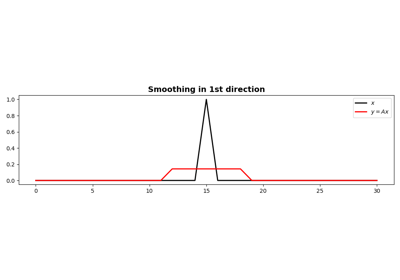1D Smoothing

1D Smoothing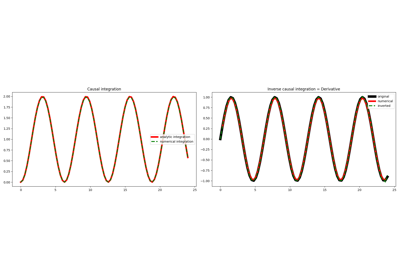Causal Integration

Causal Integration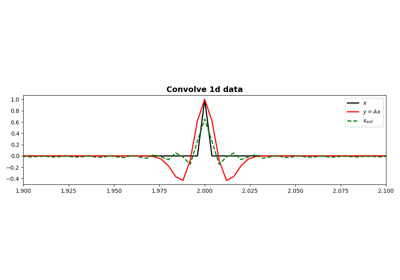Convolution

Convolution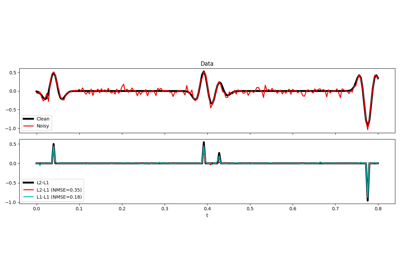L1-L1 IRLS

L1-L1 IRLS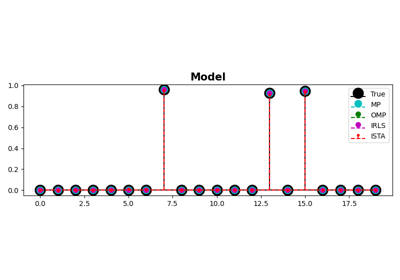MP, OMP, ISTA and FISTA

MP, OMP, ISTA and FISTA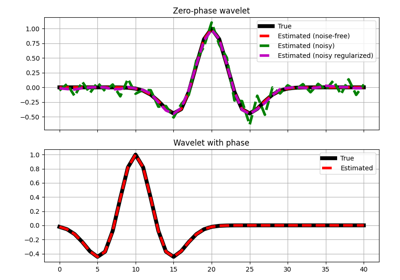Wavelet estimation

Wavelet estimation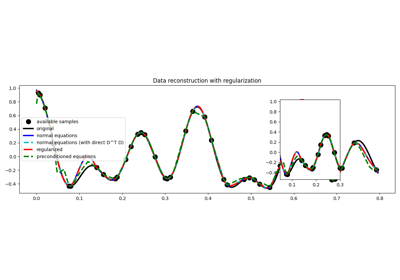03. Solvers

03. Solvers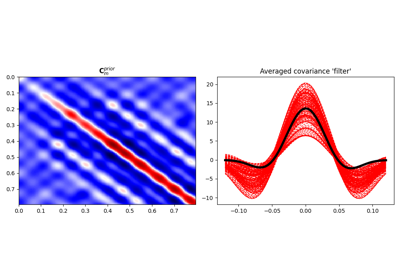04. Bayesian Inversion

04. Bayesian Inversion